Incorrect results are possible when the waterplane intersects interior "dry" surfaces, either in an upright or heeled condition. If the portion of a model being used for calculations contains more than the exterior hull, and the waterplane intersects any of the surfaces that are dry, incorrect results may be given. In almost all cases, the program will underestimate the buoyant volume. This situation is not peculiar to Orca3D; it occurs both with programs that do surface integration like Orca3D, as well as with programs that use traditional section integration, such as GHS and others. Because Rhino makes it so easy to create complete and complex models, it is becoming a more common issue. Note that multihulls do not necessarily have this issue, as long as the waterplane only intersects "wet" surfaces.

The following figures show graphically how some models are treated by the calculations:

Sailboat Hull with Cockpit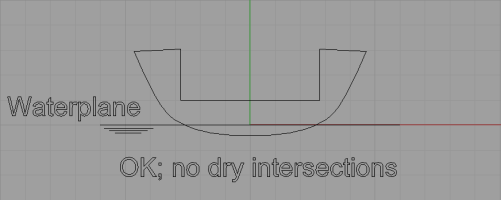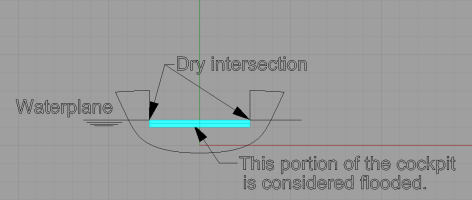Barge Hull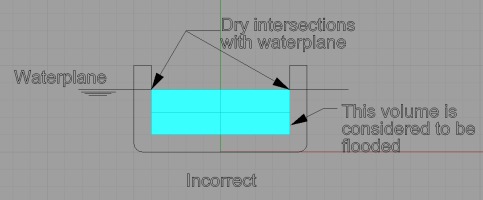Rigid Inflatable Boat (RIB)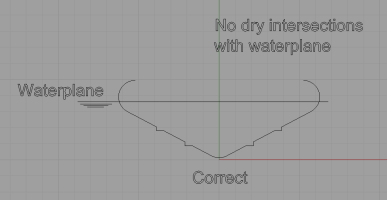Multihull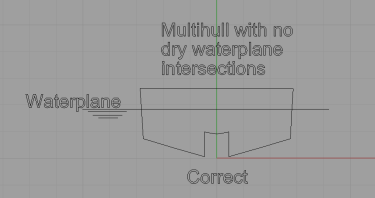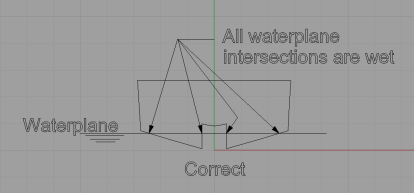When doing upright calculations, be sure to select only those surfaces that will be wet, and more precisely, do not select any surfaces that would create "dry" waterplane intersections. When running stability calculations, it may be necessary to run the calculations in multiple steps. As an example, consider the sailboat hull shown below: If the entire model is selected, and stability calculations are run to an angle such that the inside of the cockpit is intersected by the waterplane, the model will be treated as if the cockpit were flooded.The correct procedure is to run the calculations in multiple steps, with the appropriate surfaces being selected in each step as they become wetted. The use of Points of Interest will allow you to see where the cutoff angle is for each step in the calculations. In the figure above, the model has heeled to a point where there is a dry waterplane intersection. Instead, as shown in the figure below, only the hull and side deck surfaces should be selected, and a point of interest is placed at the inside corner of the side deck.The model is then run through a range of heel angles, and the resulting point of interest heights that are reported in the output are used to determine at what angle the point of interest is becoming submerged. In this example, it is seen that the point of interest becomes submerged at 45 degrees. Next, as shown in the figure below, the calculations are run again, but starting from 45 degrees, with the cockpit surfaces included.By using the option "Add Plane Representing Water Surface," you can study your model at a given heel angle to determine if you have created any dry intersections with the waterplane. If there are such intersections, you should go through the process described above in order to obtain the correct results.